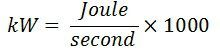# Difference Between kW & kWh

The most significant difference between the kW and kWh is that the KW measure the power generate and utilise by the devices, whereas the kWh measure the energy consumed by the electrical equipment. The other differences between kW and kWh are explained below in the comparison chart.

The word demand shows the kW, and the word consumption describes the kWh. The kW stands for kilowatt, and one kW is equal to 1000 watts. It is used for measuring the power utilised by small devices like a bulb, cooler, fan, etc. Whereas, the kWh stands for a kilowatt hour. The kWh measures the energy utilises by the electrical equipment over a given period. The kilowatt hour is mainly used for measuring the electricity bill. The one unit of kWh is equal to the 3.6 megajoules per second.

## Comparison Chart

Basis for Comparison KW KWh
Definition Measure the power induces and utilizes by the electrical devices.Measure the energy consumes by the appliances.
Full Form Kilowatt Kilowatt Hour
FormulaApplications Measure power of small appliances like, bulb, motor etc. Use for calculating the electrical energy.

### Definition of kWh

The kW measure the power delivered by the electrical devices with respect to time, or we can say that it calculates the work performed by the electrical appliances regarding time. The home appliances like the fan, electric heater, the lamp have rated power. And this power is measured in kilowatts.

### Definition of kWh

The kWh measure the work performed by the appliances at the given period.  The kWh measure the energy utilised by the machines. The small consumption of energy is measured in kilowatt-hours, and the large consumption of energy is measured in megawatt hours.

Example – Let the heater runs 24 hours of a day and consumes 1500 – 2000 watts of power. So the total energy consumed by the heater is measured by the product of time and power utilised by the heater.

Energy (in kWh ) = Power (kW) X Time (24).

Energy = 1500 x 24 /1000

Energy = 36 kWh

## Key Differences Between kW and kWh

1. The kW is the unit of measuring the power utilise and consume by electrical appliances like motor, generator, heater, etc. Whereas the kWh measure the energy consume by the electrical appliances
2. The kW stands for kilowatt, and the full form of kWh is kilowatt hour. The kWh measures the energy which is the product of the total power consumed by the devices and the time in an hour.
3. The kW measure the power consumed by the electrical appliance like a bulb, electric heater, fan, etc. The kWh is mainly used for calculating the energy utilised by the machines.

The kilowatt hour primarily uses in a billing company for measuring energy consumption.

### 1 thought on “Difference Between kW & kWh”

1. Suzie Mcgarrity

Good tidings, generally I never remark on online journals yet your article is persuading to the point that I never stop myself to say something regarding it. You’re working effectively, Keep it up.